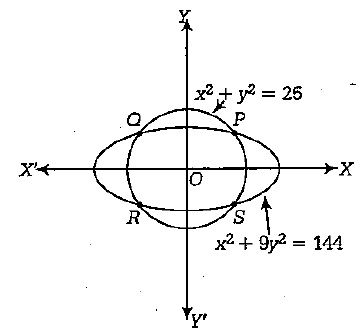# If A = [(x, y) : x2 + y2 = 25] and B = [(x, y) : x2 + 9y2 = 144], then A ∩ B contains _______ points.

If A = [(x, y) : x2 + y2 = 25] and B = [(x, y) : x2 + 9y2 = 144], then A ∩ B contains _______ points.

verifiedA = Set of all values (x, y) : x2 + y2 = 25 = 52

B = [x2 / 144] + [y2 / 16] = 1

i.e., [x/ (12)2] + [y2 / (4)2] = 1.

Clearly, A ∩ B consists of four points